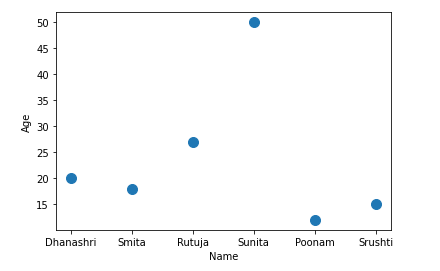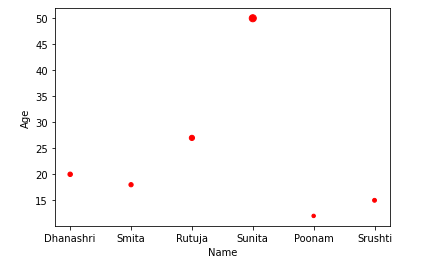# Pandas Scatter Plot – DataFrame.plot.scatter()

• Last Updated : 25 Feb, 2021

A Scatter plot is a type of data visualization technique that shows the relationship between two numerical variables. For plotting to scatter plot using pandas there is DataFrame class and this class has a member called plot. Calling the scatter() method on the plot member draws a plot between two variables or two columns of pandas DataFrame.

Syntax: DataFrame.plot.scatter(x, y, s = none, c = none)

Parameter:

x: column name to be used as horizontal coordinates for each point
y: column name to be used as vertical coordinates for each point
s: size of dots
c: color of dots

### Steps:

• Import necessary libraries.
• Prepare a data
• Convert prepared data into DataFrame
• Draw a scatter plot

Example 1: In this example, we will plot the scatter plot using dataframe, Here we will create the dataframe and plot the scatter plot using different columns.

## Python3

 `# Program to draw scatter plot using Dataframe.plot ` `# Import libraries ` `import` `pandas as pd ` ` `  `# Prepare data ` `data``=``{``'Name'``:[``'Dhanashri'``, ``'Smita'``, ``'Rutuja'``, ` `              ``'Sunita'``, ``'Poonam'``, ``'Srushti'``], ` `      ``'Age'``:[``20``, ``18``, ``27``, ``50``, ``12``, ``15``]} ` ` `  `# Load data into DataFrame ` `df ``=` `pd.DataFrame(data ``=` `data); ` ` `  `# Draw a scatter plot ` `df.plot.scatter(x ``=` `'Name'``, y ``=` `'Age'``, s ``=` `100``); `

Output:Example 2: Here we will create the dataframe and plot the scatter plot using different columns with style data points.

## Python3

 `# Program to draw scatter plot using Dataframe.plot ` `# Import libraries ` `import` `pandas as pd ` ` `  `# Prepare data ` `data``=``{``'Name'``:[``'Dhanashri'``, ``'Smita'``, ``'Rutuja'``, ` `              ``'Sunita'``, ``'Poonam'``, ``'Srushti'``], ` `      ``'Age'``:[ ``20``, ``18``, ``27``, ``50``, ``12``, ``15``]} ` ` `  `# Load data into DataFrame ` `df ``=` `pd.DataFrame(data ``=` `data); ` ` `  `# Draw a scatter plot and here size of dots determined by age of person ` `df.plot.scatter(x ``=` `'Name'``, y ``=` `'Age'``, s ``=` `'Age'``, c ``=` `'red'``); `

Output:My Personal Notes arrow_drop_up
Recommended Articles
Page :